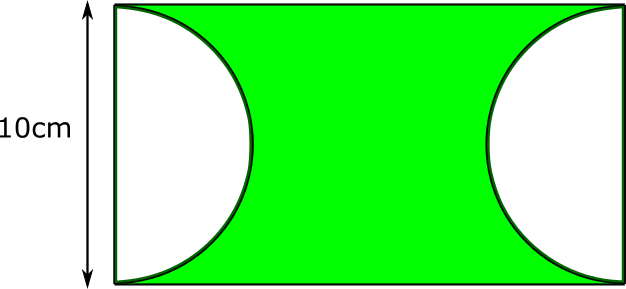#### You may also like### Some(?) of the Parts

A circle touches the lines OA, OB and AB where OA and OB are perpendicular. Show that the diameter of the circle is equal to the perimeter of the triangleA 1 metre cube has one face on the ground and one face against a wall. A 4 metre ladder leans against the wall and just touches the cube. How high is the top of the ladder above the ground?Four rods are hinged at their ends to form a convex quadrilateral. Investigate the different shapes that the quadrilateral can take. Be patient this problem may be slow to load.

# Semicircle Distance

##### Age 14 to 16 ShortChallenge Level

The diagram shows a rectangle and two semicircles.

The height of the rectangle 10 cm, and the area of the shaded region is 125 cm$^2$.

What is the shortest distance between the two semicircles?

Give your answer in terms of $\pi$.This problem is adapted from the World Mathematics Championships
You can find more short problems, arranged by curriculum topic, in our short problems collection.